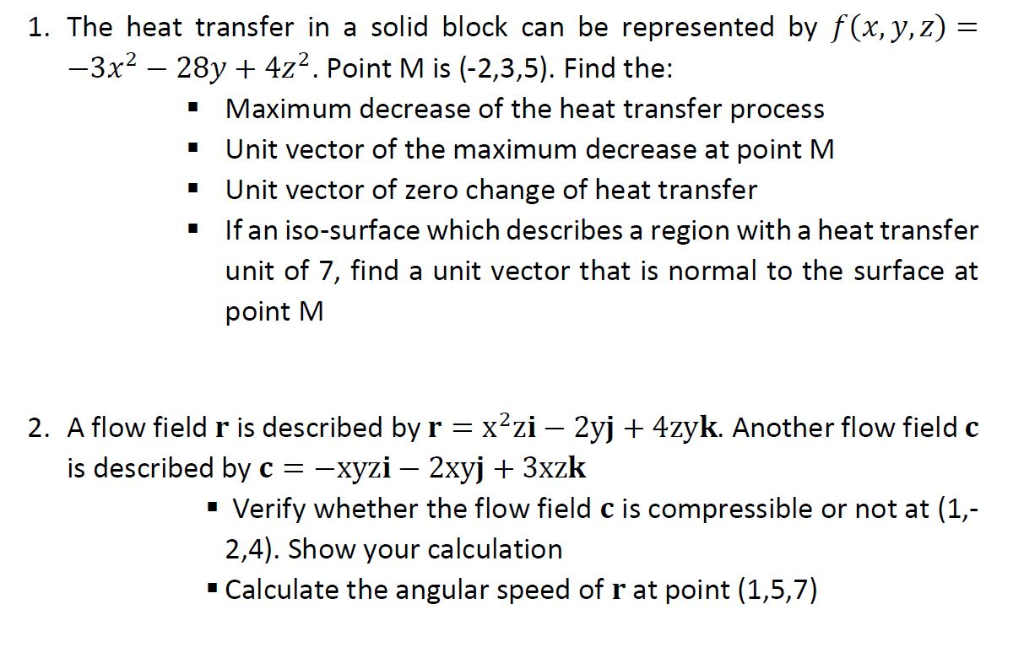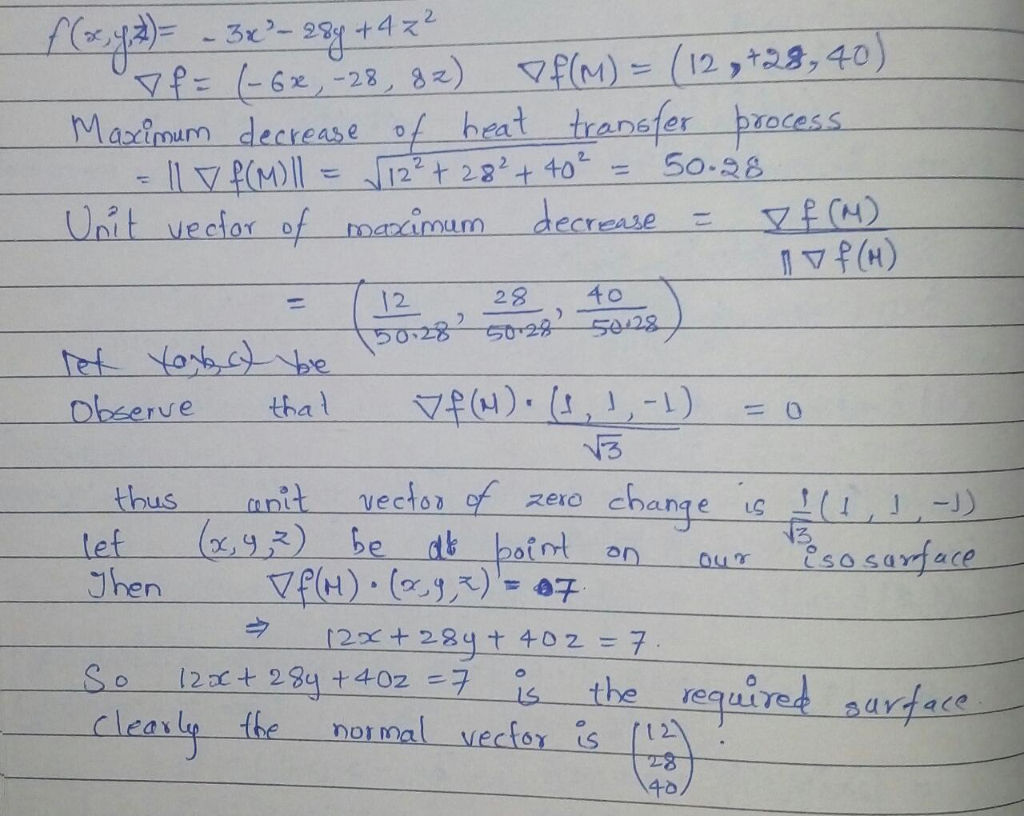# 1. The heat transfer in a solid block can be represented by f(x, y,z)- 3x2 28y 4z2. Point M is (-2,3,5). Find the: Maxi...1. The heat transfer in a solid block can be represented by f(x, y,z)- 3x2 28y 4z2. Point M is (-2,3,5). Find the: Maximum decrease of the heat transfer process " Unit vector of the maximum decrease at point M Unit vector of zero change of heat transfer If an iso-surface which describes a region with a heat transfer " " " unit of 7, find a unit vector that is normal to the surface at point M 2. A flow field r is described by rx2zi - 2yj + 4zyk. Another flow field c is described by c--хул-2xy + 3xzk " Verify whether the flow field c is compressible or not at (1, 2,4). Show your calculation Calculate the angular speed of r at point (1,5,7)first complete question according to chegg policy.

##### Add Answer of: 1. The heat transfer in a solid block can be represented by f(x, y,z)- 3x2 28y 4z2. Point M is (-2,3,5). Find the: Maxi...
More Homework Help Questions Additional questions in this topic.